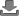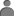clear
Keywords
clear
to
clear
Show
items
Ten Frames by Kellie Gaffney
Understand the ones and tens positions and the numerals 10-2084 - 7
07 Dec 2012
Introduction to Probability by Louis Paul
An introduction to the concepts of probabilty using dice and cards311 - 13
07 Dec 2012
Representing numbers in base ten blocks
A workbook containing thousands, hundreds, tens and ones sets of base ten blocks for representing numbers in base ten135 - 8
07 Dec 2012
Introduction to Algebra by Cristy Holtzclaw
A brief introduction to simple algebraic variables and solving simple equations210 - 12
07 Dec 2012
Finding the Area by Jaclyn Summerlin
Students learn techniques to find the area of squares, rectangles and similar shapes, as well as design shapes for a given area85 - 10
07 Dec 2012
Perimeter
Students find the perimeter of various polygons45 - 10
07 Dec 2012
Finding the Derivative of Sine by Brian Leyhane
Students learn how to find the derivatives of 8 trigonometric functions113 - 16
07 Dec 2012
Comparing and Ordering Fractions by Brenda Ballie
Learn to order and compare fractions and appy knowledge of least common denominator to order fractions138 - 12
06 Dec 2012
The Soccer Challenge by Russell Bates
A soccer themed game for two teams. Tests basic subtraction skills125 - 10
05 Dec 2012
Pythagorean Theorem - Theory and Practise
Brief introduction to the pythagorean equation and ten practise questions complete with worked answers29 - 15
04 Dec 2012
Show
items